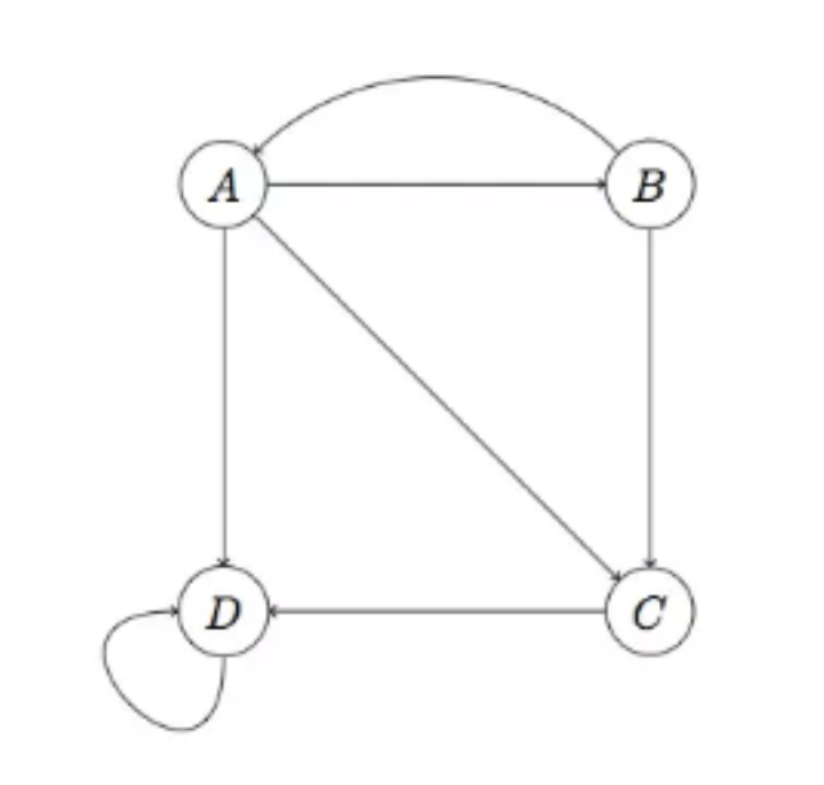PageRank 算法

## 链接预测简介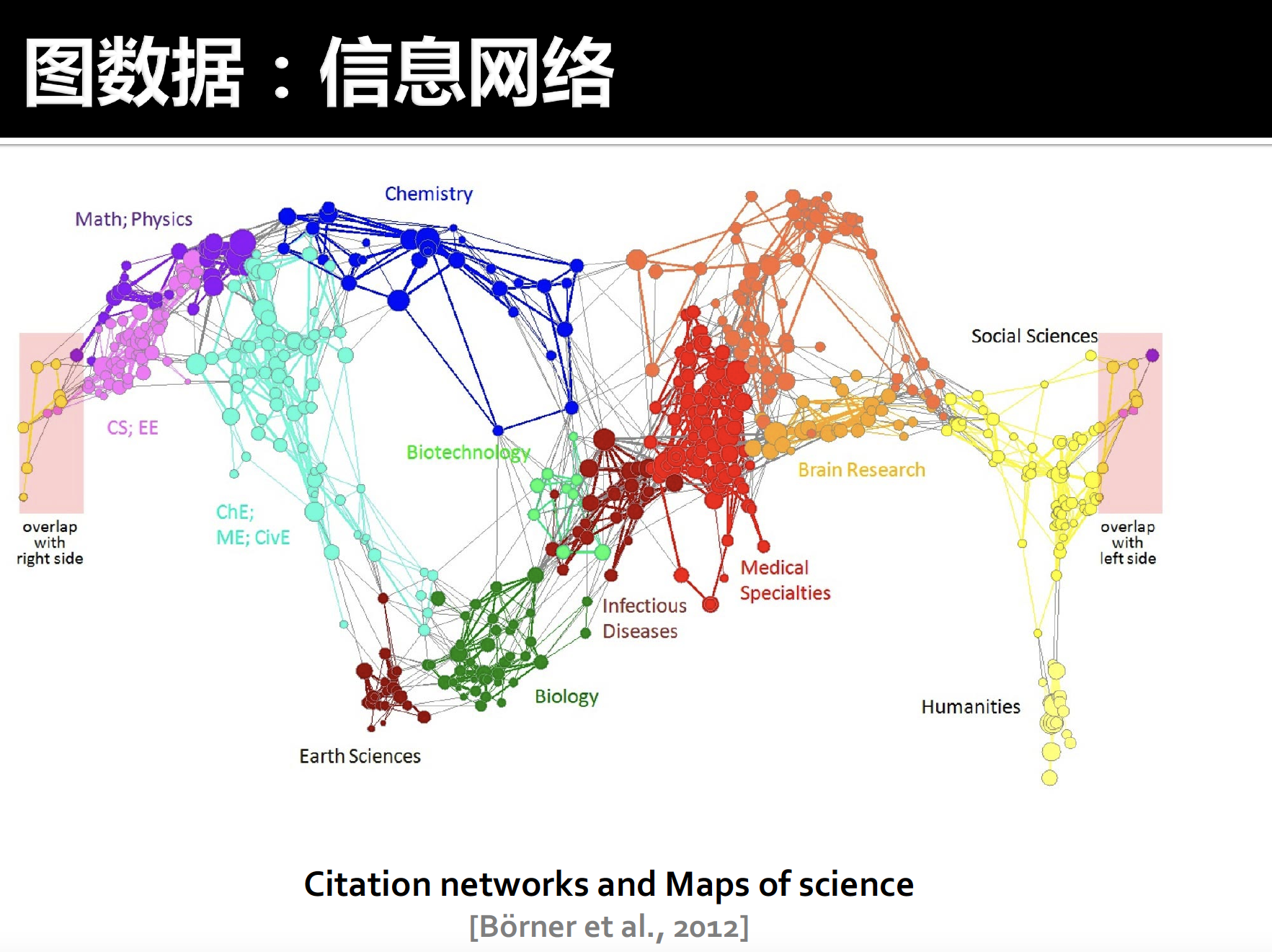## 网页数据

• 节点：网页
• 边：超链接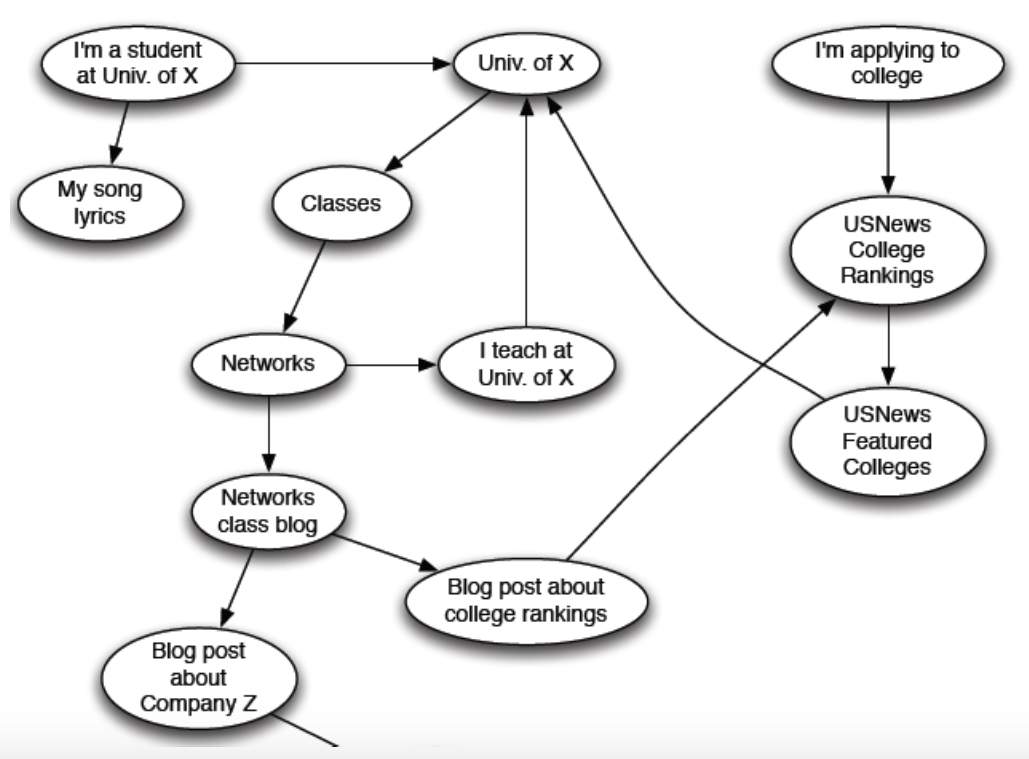1. 网络中存在很多回答，到底应该相信哪个回答？
2. 查询“数据”的最佳的回答是什么

## PageRank 算法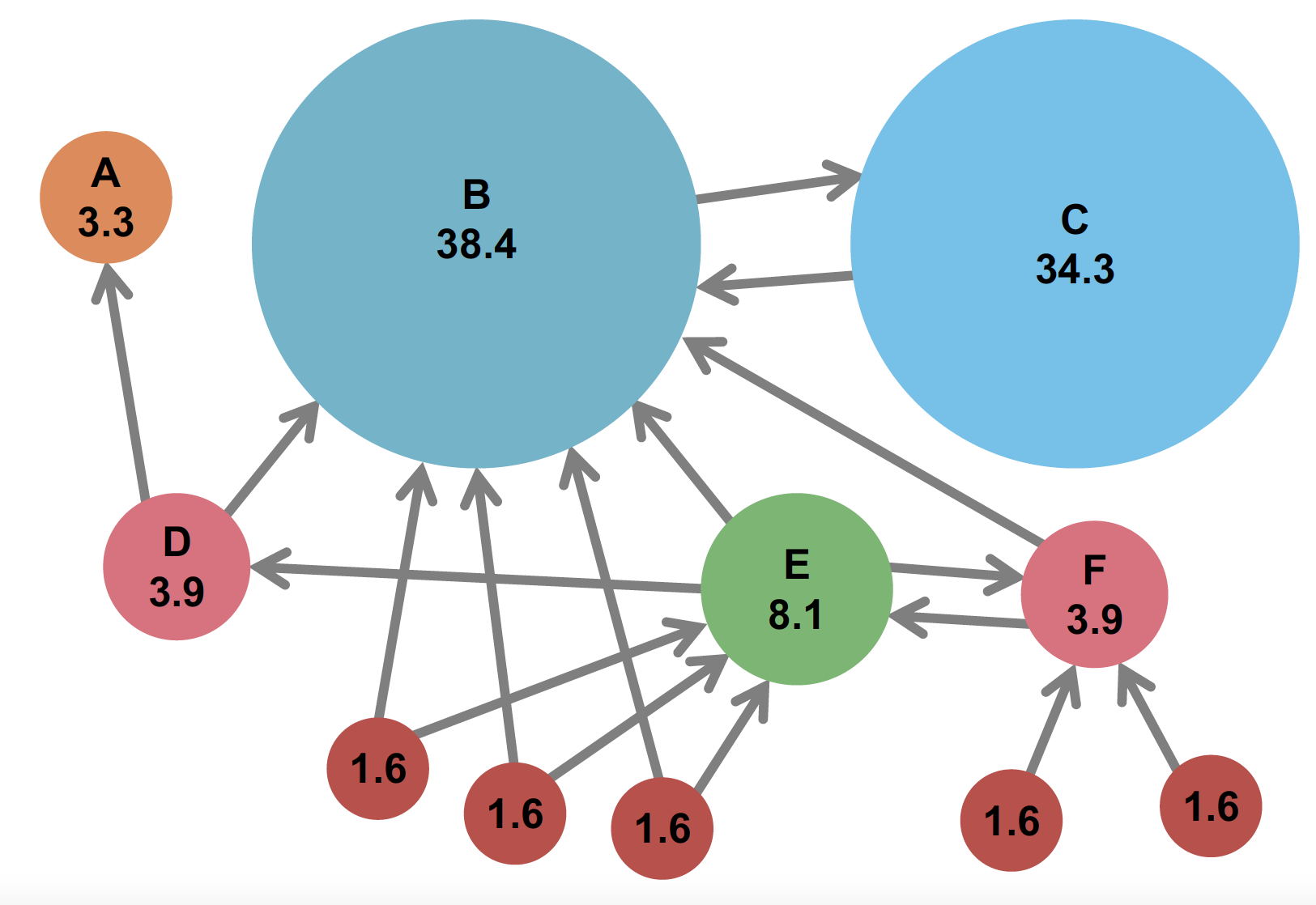1. 计算转移矩阵：转移矩阵 $M$ 是一个 $n \times n$ 的矩阵，$m_{ij}$ 定义为从 $j$ 网页流向 $i$ 网页占 $j$ 网页的所有出链的比例（即 $\frac{j \rightarrow i 的路径数量}{j 的所有出链数量}$）。
1. 易知，转移矩阵 $M$ 的每一列的和为 1
2. 转移矩阵 $M$ 的每一个元素的范围都在 $[0, 1]$ 之间
2. 迭代的计算链接评分，$PR(i+1) = M PR(i)$（其中 $PR(0) = \frac{ee^T}{n}$$n$ 为节点数量）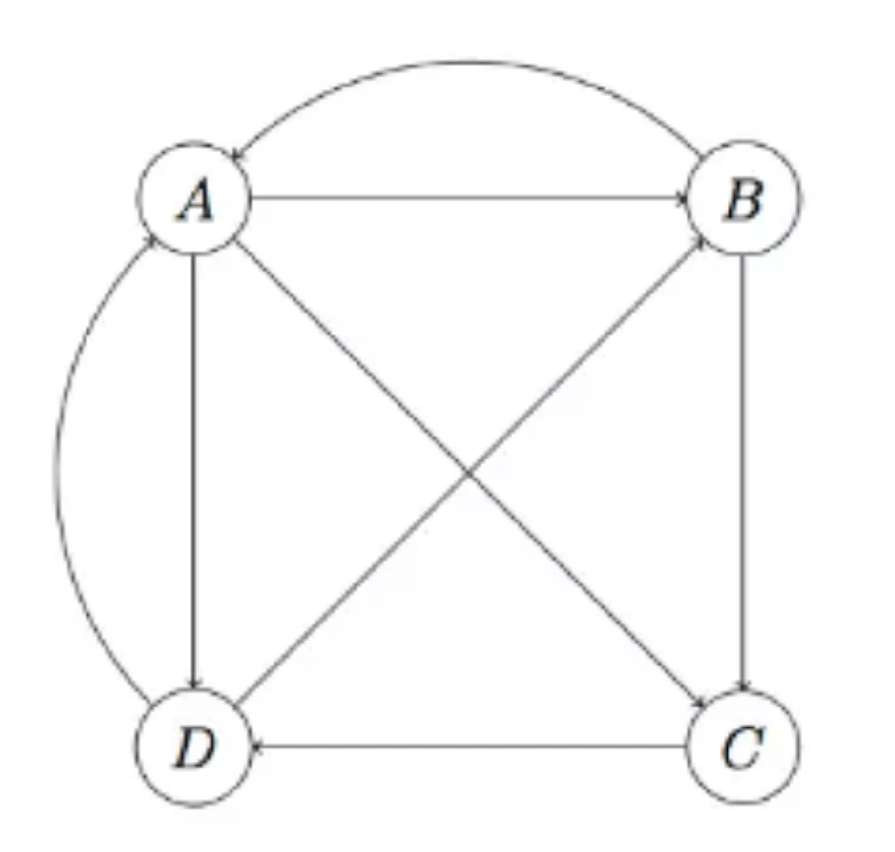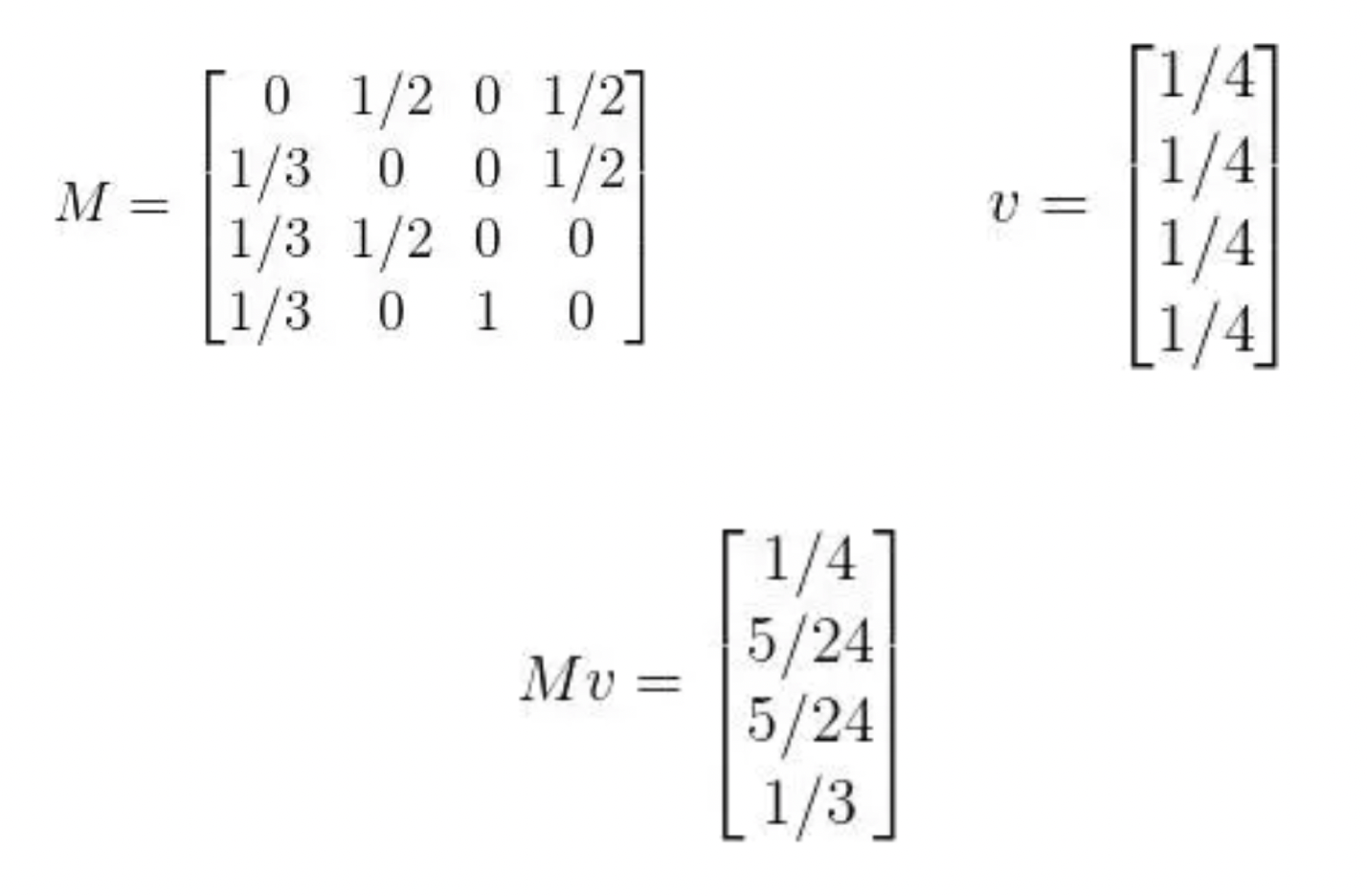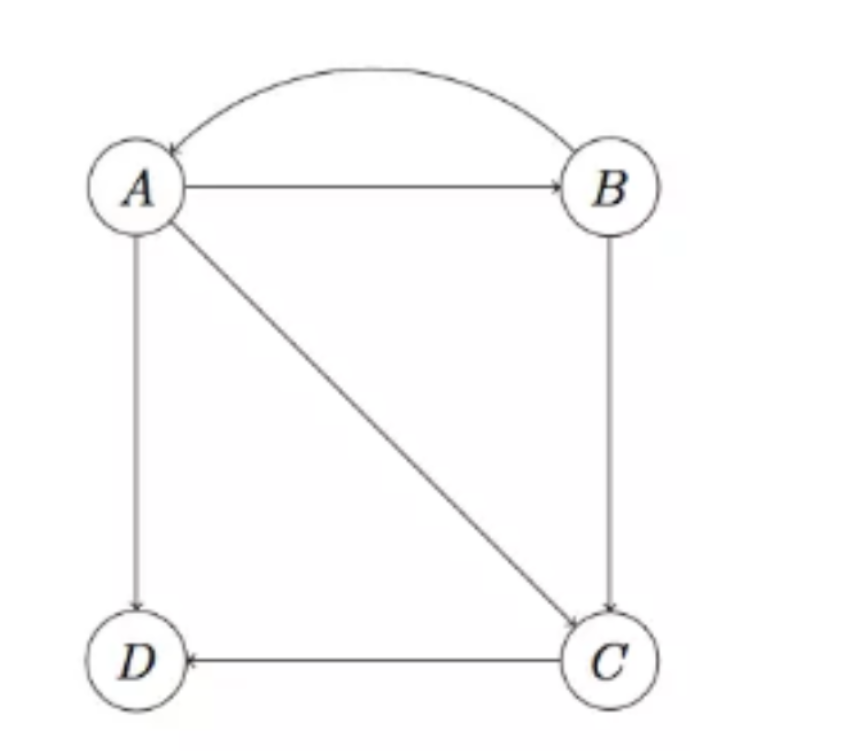### Spider Trap 问题